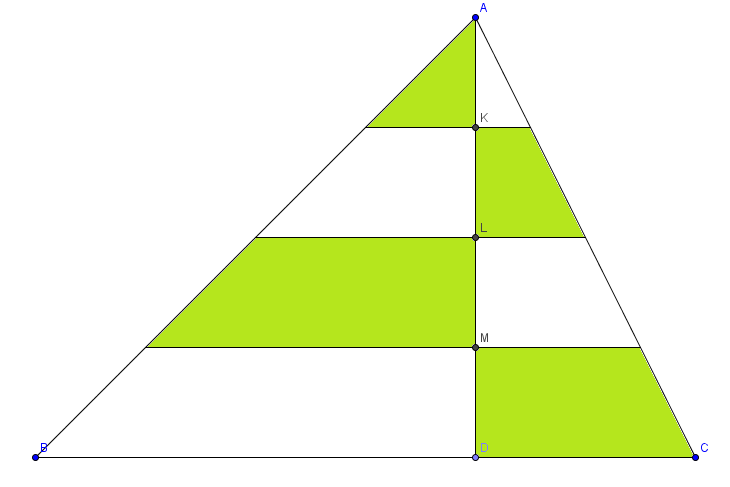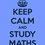# RMO 2014 Delhi Region Q.1Let $ABC$ be a triangle and let $AD$ be the perpendicular from $A$ on to $BC$. let $K,L,M$ be the points on $AD$ such that $AK=KL=LM=MD$. If the sum of the areas of the shaded regions is equal to the sum of the unshaded regions, prove that $BD=DC$.

• You can find rest of the problems here

• You can find the solutions hereNote by Aneesh Kundu
6 years, 7 months ago

This discussion board is a place to discuss our Daily Challenges and the math and science related to those challenges. Explanations are more than just a solution — they should explain the steps and thinking strategies that you used to obtain the solution. Comments should further the discussion of math and science.

When posting on Brilliant:

• Use the emojis to react to an explanation, whether you're congratulating a job well done , or just really confused .
• Ask specific questions about the challenge or the steps in somebody's explanation. Well-posed questions can add a lot to the discussion, but posting "I don't understand!" doesn't help anyone.
• Try to contribute something new to the discussion, whether it is an extension, generalization or other idea related to the challenge.

MarkdownAppears as
*italics* or _italics_ italics
**bold** or __bold__ bold
- bulleted- list
• bulleted
• list
1. numbered2. list
1. numbered
2. list
Note: you must add a full line of space before and after lists for them to show up correctly
paragraph 1paragraph 2

paragraph 1

paragraph 2

[example link](https://brilliant.org)example link
> This is a quote
This is a quote
    # I indented these lines
# 4 spaces, and now they show
# up as a code block.

print "hello world"
# I indented these lines
# 4 spaces, and now they show
# up as a code block.

print "hello world"
MathAppears as
Remember to wrap math in $$ ... $$ or $ ... $ to ensure proper formatting.
2 \times 3 $2 \times 3$
2^{34} $2^{34}$
a_{i-1} $a_{i-1}$
\frac{2}{3} $\frac{2}{3}$
\sqrt{2} $\sqrt{2}$
\sum_{i=1}^3 $\sum_{i=1}^3$
\sin \theta $\sin \theta$
\boxed{123} $\boxed{123}$

Sort by:

the question is incomplete as it must be specified whether the horizontal lines are parallel

- 6 years, 6 months ago

Assume that they are parallel

- 6 years, 6 months ago

i got 3 correct, and attempted 5 in delhi rmo what chance do i have to get selected

- 6 years, 7 months ago

The difference of the area of the circumscribed n the inscribed square of a circle is 35 sq.m.Find the area of the circle!!!!!!!!!!!

- 6 years, 7 months ago

It must be isoceles or equilateral triangle, AB must be equal to AC

- 6 years, 7 months ago

this tym's rmo was the easiest..........and it took me some time to believe that this was a rmo question

- 6 years, 7 months ago

There are 4 similar triangles if you observe

let BD = a and DC = b

Observe the left side of the figure

The segment above BD will be 3/4 of a

the segment above will be 2/4 of a

and the topmost segment will be 1/4 of a

Similarly the lengths of segments on the right can be found Let AK = h,

Use the formula for area of trapezium and area of triangle, you will get a = b

- 6 years, 7 months ago

assume all lines from the points K,L,M are parallel to the base. Let DC = k*BD ; Name the points on the side AB formed by intersection of parallel lines through K,L,M as - K1 ,L1 ,M1 and for the side AC the points of intersection as K2 , L2 ,M2 . Let the area of triangle AK1K be A1, and the area of the trapezium below it (KK1L1L) be A2, area of trapezium LL1M1M be A3, area of trapezium MM1BD be A4;

"The area of the triangles and trapeziums on the right will be 'k' times the area of the triangles on the left" Since we have assumed that DC = k*BC and the lines are parallel to the base

So area of triangle on the right say A1' = k* A1; area of right trapezium below (KK2L2L) = A2' = kA2; let area of trapezium on the right LL2M2M = A3' = kA3; area of trapezium MM2CD = (say) A4' = k*A4;

Given that area of shaded region = area of unshaded region implies (shaded) A1 + A2' + A3 + A4' = A1' + A2 + A3' + A4 (unshaded) .......equation(1)

"In triangle ABD area of all the trapezium's are proportional to the area of top triangle AK1K " Let area of trapezium KK1L1L = A2 = xA1 ; (x is the proportionality constant) area of trapezium LL1M1M =A3 = y A1 ; area of trapezium MM1BD = A4 = z*A1;

Hence A2' = kxA1 (since A2' = kA2 and A2 = xA1) Similarly A3' = kyA1 (since A3' = kA3 and A3 = yA1)
similarly A4' = kzA1;

substitute A2, A3, A4 , A1' ,A2', A3', A4' in terms of A1 in equation 1....

A1 + A2' + A3 + A4' = A1' + A2 + A3' + A4

    A1 + k*x*A1 + y*A1 +  k*z*A1 = k*A1 + x*A1 + k*y*A1 + z*A1


=> 1 + kx + y + kz = k + x + ky + z (dividing by A1 which is non zero) => (1-k) + x(k-1) + y(1-k) + z(k-1) = 0 => -(k-1) + x(k-1) -y(k-1) + z*(k-1) = 0 => (k-1)(x - y + z -1) = 0 => k = 1;

we assumed DC = k*BD => DC = BD
Hence proved...

- 6 years, 7 months ago

I'm not a math wizard but I presume the figure is an equilateral triangle?

- 6 years, 7 months ago

No, it's actually any general triangle, but with parallel lines cutting it into several pieces.

- 6 years, 7 months ago

it makes no difference whether the lines are horizontal or not. if the sum of the areas of corresponding sides are equal and the heights are equal, abd and adc are congruent, so their bases bd and dc are equal

- 6 years, 7 months ago

to prove that BD=DC, you must think of the drawing as an isosceles triangle, without arguing or just ignore its unsymmetrical form. And if you will reconstruct the drawing to an isosceles triangle, only thus you can prove that BD=CD.

- 6 years, 7 months ago

simply.. equate the area of shaded and unshaded region and then apply the property of similar triangles..

- 6 years, 7 months ago

Isn't the prependicular from A is its median,,,,,if it is so,,,,,,,,then BD=DC

- 6 years, 7 months ago

If the areas of the shaded and unshaded parts are equal, then that means that 1/2 of the triangle is shaded and the other half is not. if we try to arrange the triangle such that the half is shaded and the other other half is not, we will notice that they share the same measures of altitudes.. and so for their areas to be totally equal the measures of the bases of the two triangles must be equal as well.. which are BD and DC..

- 6 years, 7 months ago

Make it to a rectangle or square.square ll be formed if it is a equilateral triangle . Isosceles triangle forms a rectangle.( shaded and unshaded regions allign with their respective kind forming rectangles. Two shaded n two unshaded rectangles of equal area ll be fromed.

- 6 years, 7 months ago

what if we drop 3 more perpendiculars from the corners of trapeziums and triangles?....we'll get equal squares.

- 6 years, 7 months ago

That is a special case and ocurrs only when $BD=\dfrac{AD}{3}$

- 6 years, 7 months ago

if we look at triangles - (area of green triangle) by side AK... then its ratio with the area of triangles ABD and and ACD is 1:16 as AK:AD :: 1:4 ... thus it is possible iff... area of ABD = area of ACD....as they have a common perpendicular... so it also means there bases are equal

- 6 years, 7 months ago

Area of the green ∆ is a quarter of ADB. But we cant say the same for ADC, for that we first need to show that $BD=DC$ which is exactly what we needed to prove.

- 6 years, 7 months ago

on the other hand ,the areas of shaded & unshaded region is equal(in given fig.) iff the triangle is Isosceles triangle& equilateral otherwise area must not be same(referring given fig)

- 6 years, 7 months ago

I have solved it using simple geometry of similar triangles...

- 6 years, 7 months ago

By therom of similar triangles, all horizontal lines are parallel. You have just to use formula Area of triangle is half base x height and Area of trapezium is 1/2(sum of parallel sides x height)and you get the solution.

- 6 years, 7 months ago

It is an intuitively obvious result. To prove this, - understand that the result is certainly true when BD = DC. - take any point D' on BC and prove that for the regions formed between AD and AD', the shaded regions do not equal the unshaded regions in area. It is simple then.

- 6 years, 7 months ago

when I was giving the test in Narendrapur Ramkrishna Mission(my test centre) a correction was given from the co-ordinator which said that the lines were parallel.

- 6 years, 7 months ago

i have solved it in 2 min and it was so eassy

- 6 years, 7 months ago

How did u solve Tiwari ji

- 6 years, 7 months ago

HOW DID YOU SOLVE IT? CAN YOU EXPLAIN ME?

- 6 years, 7 months ago

its not given in the question paper that the lines are parallel, i think instead we had too think the contrary that if lines arent parallel then what???? thats the real question !!! otherwise the question can be done by a 7 standard student! i am waitin for the official sol. to know whats the correct answer

- 6 years, 7 months ago

In kolkata, i.e. west bengal region.......professors of ISI came and corrected the problem......and this was what they gave...........u aren't alone bro......evn I was thinking in that line

- 6 years, 7 months ago

First of all the question was asked on the basis of the diagram provided. If the lines are not parallel then what if they intersect inside the triangle. If yes then they've not told us what the shaded and unshaded region actually is.

- 6 years, 7 months ago

is my answer lil higher levelled ?

- 6 years, 7 months ago

Can be proved by concept of similarity of area of triangle.

- 6 years, 7 months ago

Not equal

- 6 years, 7 months ago

these lines are not parallel

- 6 years, 7 months ago

Why?

- 6 years, 7 months ago

Ab=ac

- 6 years, 7 months ago

Good observation

- 6 years, 7 months ago

Easily solved, just putting the formulae for areas, it is proved.

- 6 years, 7 months ago

Its parallel :)

- 6 years, 7 months ago

I hope it is :)

- 6 years, 7 months ago

it is given that they are parallel in the question paper :)

- 6 years, 7 months ago

Did the coordinators tell that? Cause we weren't .

- 6 years, 7 months ago

In Maharashtra and Goa region paper they were given parallel.

- 6 years, 7 months ago

That's great!!!

- 6 years, 7 months ago

I solved it using trigonometry

- 6 years, 7 months ago

Same here. Took me less than a minute to solve.

- 6 years, 7 months ago

Are those three lines parallel to BC? If parallel,proof is direct!If not,what?

- 6 years, 7 months ago

Yeah, they are parallel. Actually here, this correction was made. Even there was a small correction in Problem no. 3

- 5 years, 6 months ago

I assumed them to be parallel

- 6 years, 7 months ago

The diagram in the question paper looked exactly the same.

- 6 years, 7 months ago

Well,I did for parallel and 'disproved' for non-parallel( Hope it does good)

- 6 years, 7 months ago